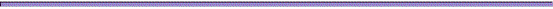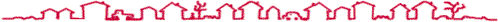Compression and Indexing
of
Massive Data Sets

## November 2002General Informations:

• Teacher: Prof. Paolo Ferragina, Dipartimento di Informatica, Pisa (ferragina@di.unipi.it).
• Lectures: 21 hours.Goal: Introduce the principles and the computational problems arising in the design of algorithms and data structures for the processing of massive data sets. In particular we will focus on string-matching problems on large text collections and discuss basic and advanced compression algorithms and indexing data structures for their processing, querying and retrieval.Exam:   Lecture notes or software project

Assignments:

• ...... [to be decided, yet]....Book references:

[MG]  Managing Gigabytes. I.H. Witten e A. Moffat e T.C. Bell. Morgan Kaufmann, 1999.

[S]   Data compression: The complete reference. D. Salomon. Springer, II edition, 2000.

(…. articles brought to class by the teacher....)List of the arguments:

1. Introduction

• Explosion of the information
• Models of computation: RAM vs. External-memory model
• Compression vs. Indexing: a paradox ?

2. Text compression

• Some impossibility results
• Background:
• Stocastic sources: Markov sources, Finite memory models
• Entropy: definition and properties
• Uniquely decipherable codes and Prefix-free codes
• Shannon's Theorem on "the noiseless channel"
• The Kolmogorov complexity of a string
•  Shannon vs. Kolmogorov.
• Huffman's algorithm:
• Huffman tree
• Canonical code
• Byte-aligned and tagged code for string-matching algorithms

• Arithmetic algorithm:
• Properties and algorithmic structure
• Time and compression-ratio performance
• Implementation
• Bzip algorithm:
• The Burrows-Wheeler transform
• The other steps: MTF, RLE and Arithmetic/Huffman
• Time and compression-ratio performance

2. Text indexing and searching

• A powerful pattern-matching tool: AGREP
• The algorithm and its complexity
• The k-errors problem
• How to use it to search compressed files: CGREP
• The Inverted Lists
• Their structure and properties: Dictionary and Postings Lists
• Variable-length encoding of postings: Unary, Gamma, Delta and Byte-aligned
• An orthogonal approach to "postings compression": Glimpse
• A powerful index: The Suffix Array
• Definition and its properties
• A simple pattern-search algorithm: O(p (occ + log n)) time complexity
• Reaching the optimal bound: O(p + occ) time complexity
• The k-mismatches problem
• Word-based suffix array
• Constructing a large suffix array
• I/O-bottlenecks in using Suffix Arrays on large data sets.
• A powerful external-memory index: The String B-tree
• Definition and its properties
• Searching for an arbitrary pattern with an optimal I/O-bound
• Engineering the data structure to reduce its space occupancy
• Combining compression and indexing: The FM-index
• Definition and its properties: a self-indexing tool
• Space occupancy proportional to the kth order entropy of the indexed text
• Counting and locating the pattern occurrences
• Word-based FM-index
• Beyond the pattern-matching: Similar set retrieval
• The problem
• Min-wise independent permutations
• Hamming space and simplex codes
• Filter indexes: an example